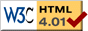# Math Refresher

previous next

Here is a quick math refresher on calculus and trig, to help you enjoy the math behind the physics simulations at MyPhysicsLab.

## Derivatives

The notation for the first derivative of a function x(t) , with respect to the variable t , can be written as

x'
x'(t)
ddt  x(t)

These are all equivalent. The notation for the second derivative is x'' or x''(t) .

Here are some of the basic rules for calculating derivatives. In the following k and n are real non-zero constants. And h(t), g(t) are functions of t .

First consider powers of t . The general rule is

ddt  t n = n t n − 1      for any n ≠ 0

Here are some examples of derivatives of powers, using the above rule:

ddt  t = 1
ddt  t 2 = 2 t
ddt  (t 3 + t 2 + t + 1) = 3t 2 + 2t + 1
ddt  1t = ddt  t −1 = − t −2 = −1t 2

Here are some basic rules about derivatives:

ddt  k = 0      (k = constant)
ddt  (k h(t)) = k  ddt h(t)      (k = constant)
ddt  (h(t) + g(t)) = ddt h(t) + ddt g(t)
ddt  (h(t) × g(t)) = h×g' + h'×g      The product rule

Here are derivatives of some very important special functions

ddt sin(t) = cos(t)
ddt cos(t) = −sin(t)
ddt e t = e t
ddt ln(t) = 1t      Natural logarithm

The all-important chain rule lets us take the derivative of functions of functions (also called function composition):

ddt h(g(t)) = h'(g(t)) × g'(t)      The chain rule

It is important to get good at using the chain rule. Here are some examples of the chain rule in action:

ddt  sin(h(t)) = cos(h(t)) h'(t)
ddt  sin(t2) = 2 t cos(t2)
ddt  e h(t) = h'(t) e h(t)
ddt  e k t = k e k t      (k = constant)
ddt  e t2 = 2 t e t2
ddt  ln(h(t)) = h'(t) ⁄ h(t)

 d⁄dt  ( 1 )  = − h'(t) h(t) h(t)2

The quotient rule gives us the derivative of a ratio of functions:

 d⁄dt  ( h(t) )  = g h' − h g' The quotient rule g(t) g2

Using the chain rule and the product rule we can derive the quotient rule:

ddt  h(t)g(t) = ddt  (h × 1g) = h' × (1g) + h × (g'g2) = (g h'h g') ⁄ g2

## Trig Identities

First, a note on some confusing notation: an exponent of −1 on a trig function means the inverse of that function (not the reciprocal!). Therefore

tan−1(x) = arctan(x)

while

tan2(x) = (tan(x))2

The best way to get comfortable with trigonometry is to think in terms of the unit circle. Most of these identities then become obvious.

sin(−x) = −sin x
cos(−x) = cos x
tan(−x) = −tan x
sin x = cos(π2x)
cos x = sin(π2x)
sin(0) = 0
cos(0) = 1
sin(π2) = 1
cos(π2) = 0
sin(π) = 0
cos(π) = −1
sin(3 π2) = −1
cos(3 π2) = 0
sin(x + 2 nπ) = sin x      n an integer
cos(x + 2 nπ) = cos x      n an integer

The famous pythagorean theorem gives us the following identity

cos2x + sin2x = 1

The sum of angles formulas are

cos(x + y) = cos x cos y − sin x sin y
cos(xy) = cos x cos y + sin x sin y
sin(x + y) = sin x cos y + cos x sin y
sin(xy) = sin x cos y − cos x sin y

This web page was first published April 2001.

previous next